• Weather
• Ocean
• Ice & Icebergs
• Other Hazards
• Archive Data
• Digital Forecasts
• Electronic Marine Charting (ECDIS)
• Environmental
• Extratropical Storm Surge Models
• Freezing Spray
• GIS
• Observations
• Ocean
• Probabilistic
• Product Information
• Receiving Products
• News
• Social Media

 Hurricane Force Warnings in Effect Atlantic:  High Seas

## GWES Probabilistic Wave Height Guidance

Use the drop down menus below to change the display.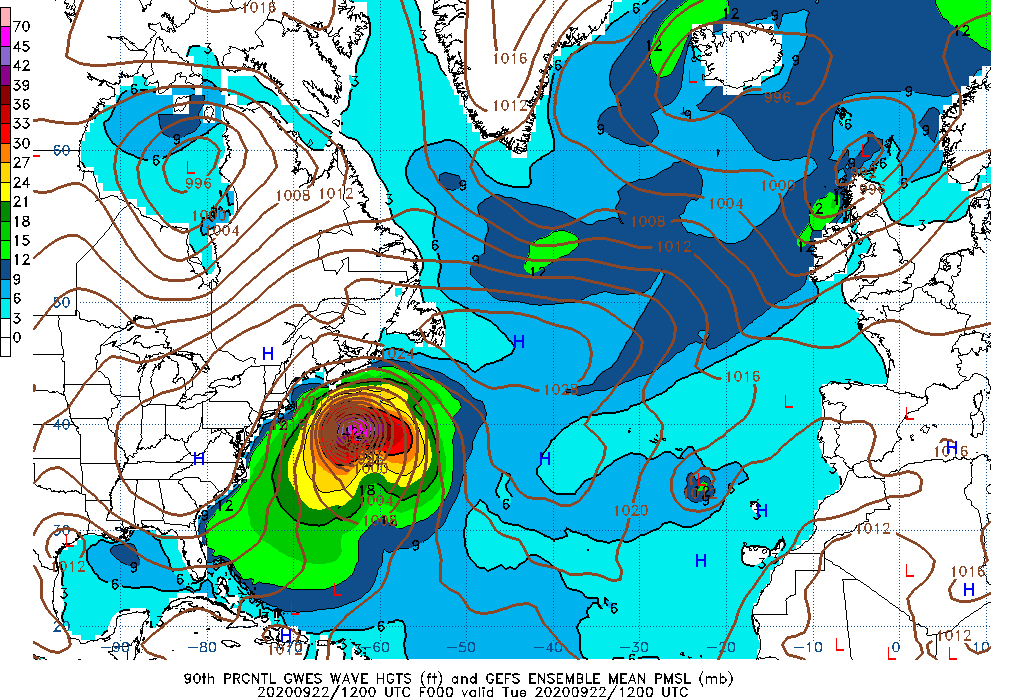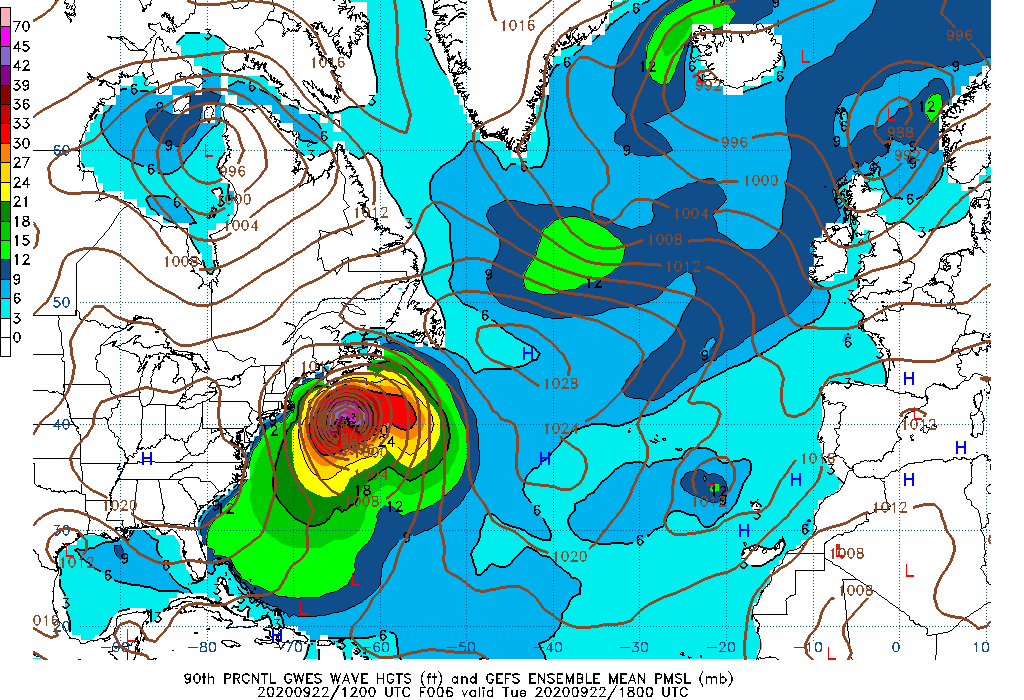000 Hour Wave Height 90th Percentile 006 Hour Wave Height 90th Percentile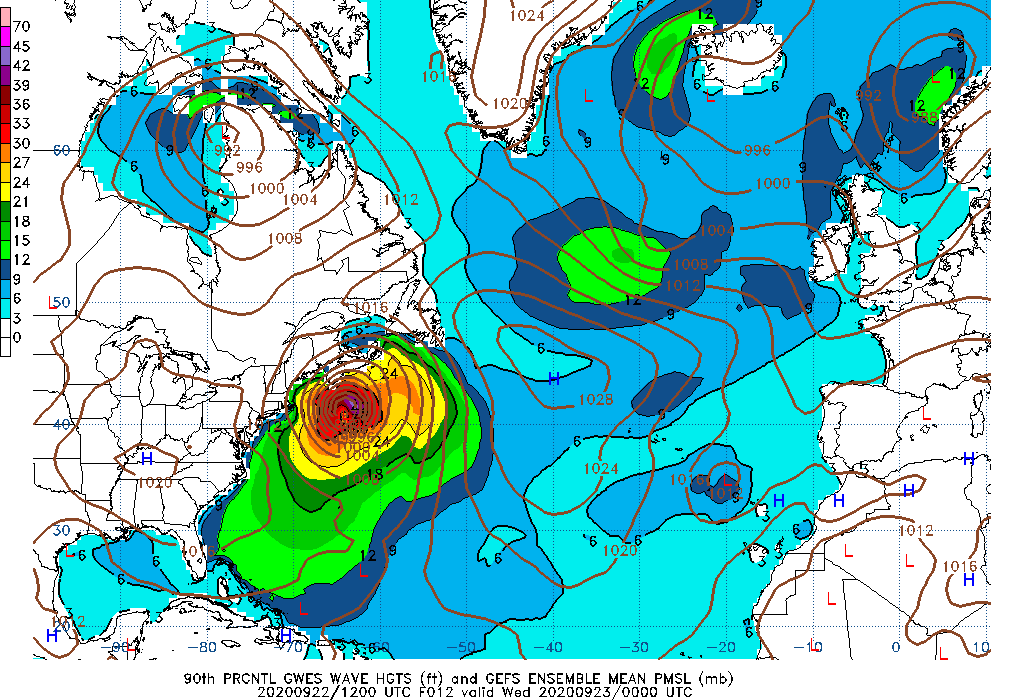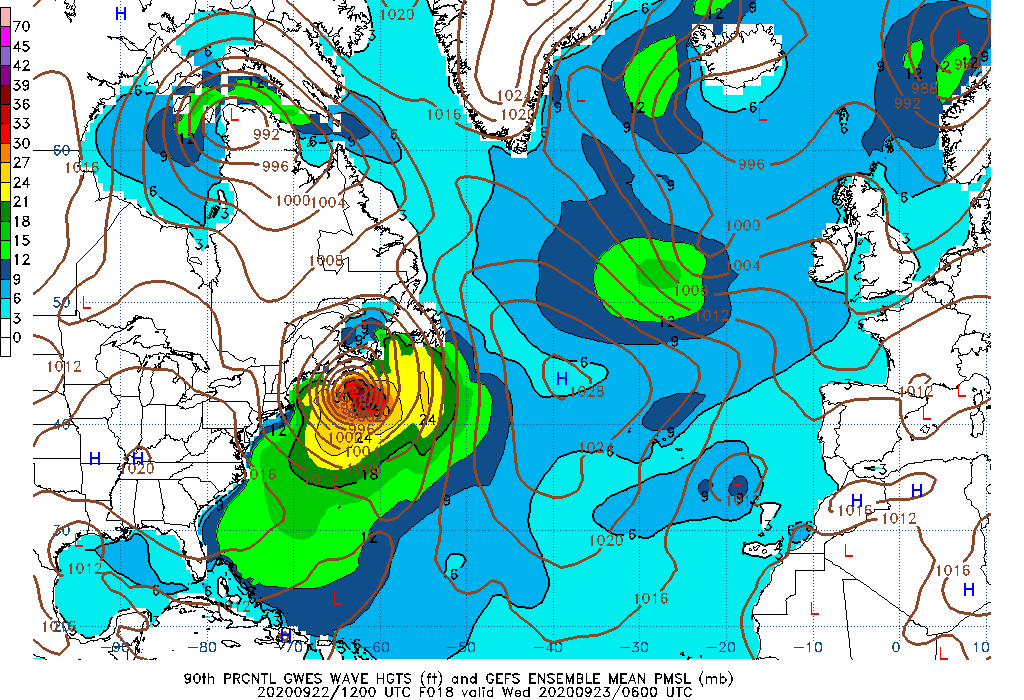012 Hour Wave Height 90th Percentile 018 Hour Wave Height 90th Percentile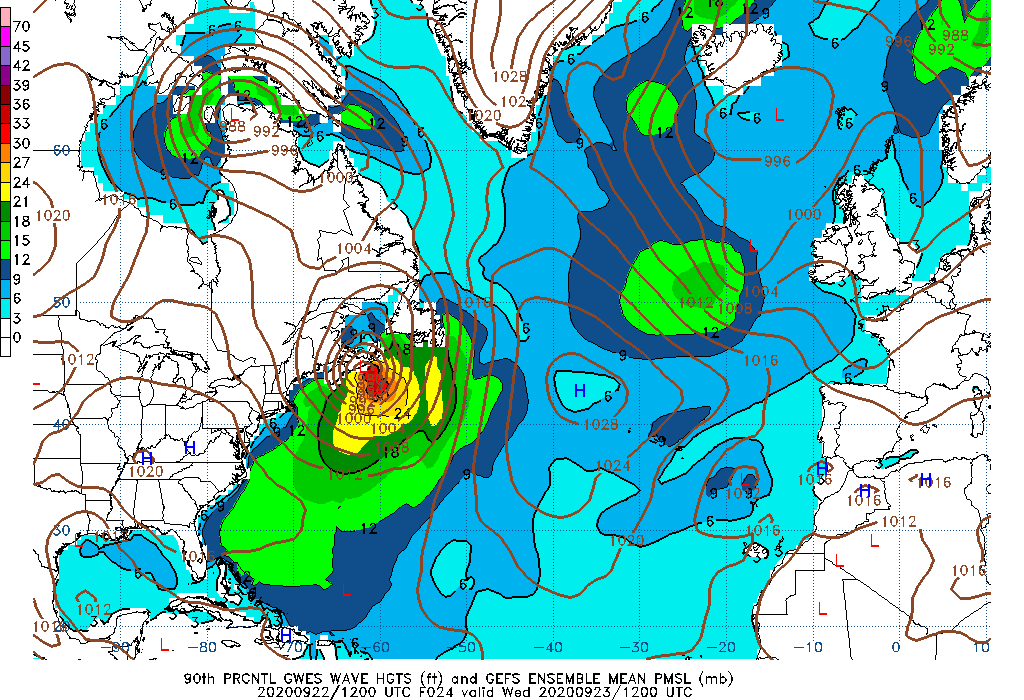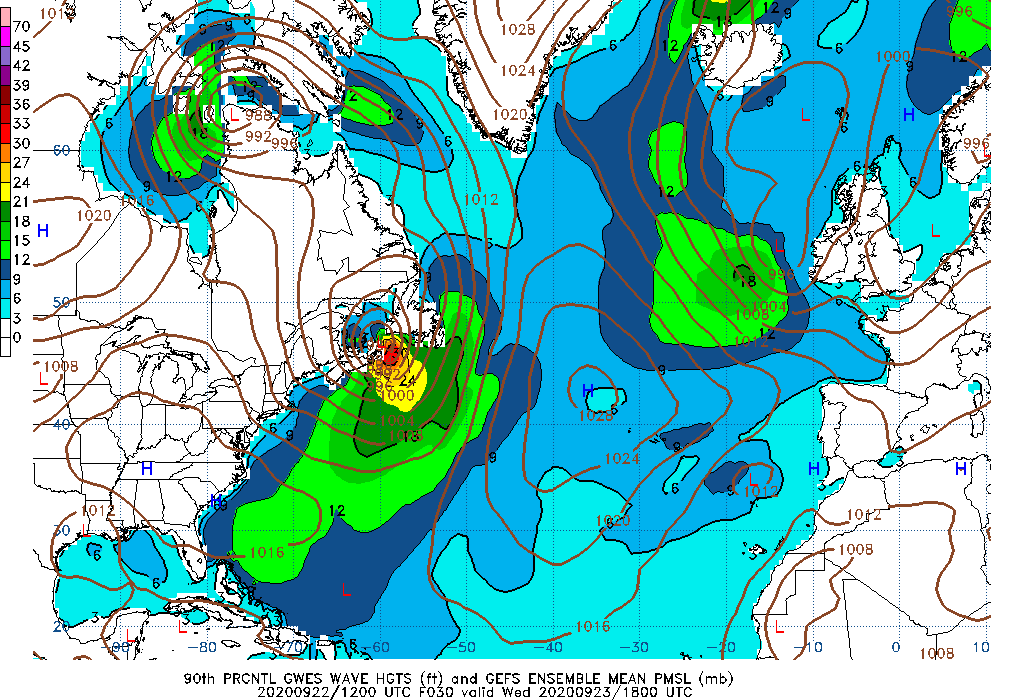024 Hour Wave Height 90th Percentile 030 Hour Wave Height 90th Percentile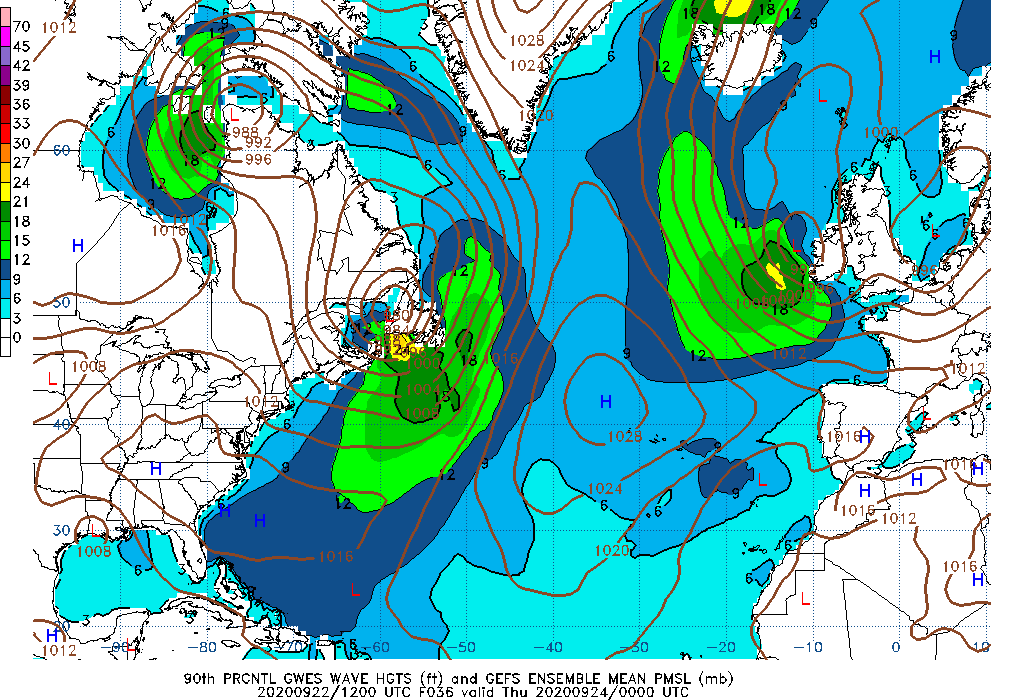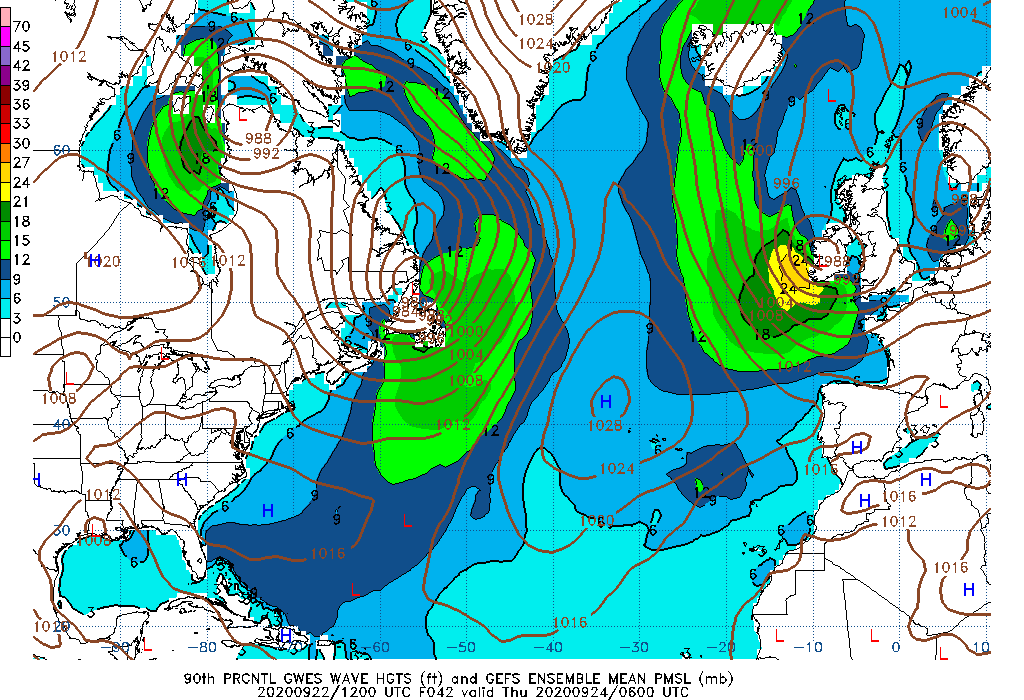036 Hour Wave Height 90th Percentile 042 Hour Wave Height 90th Percentile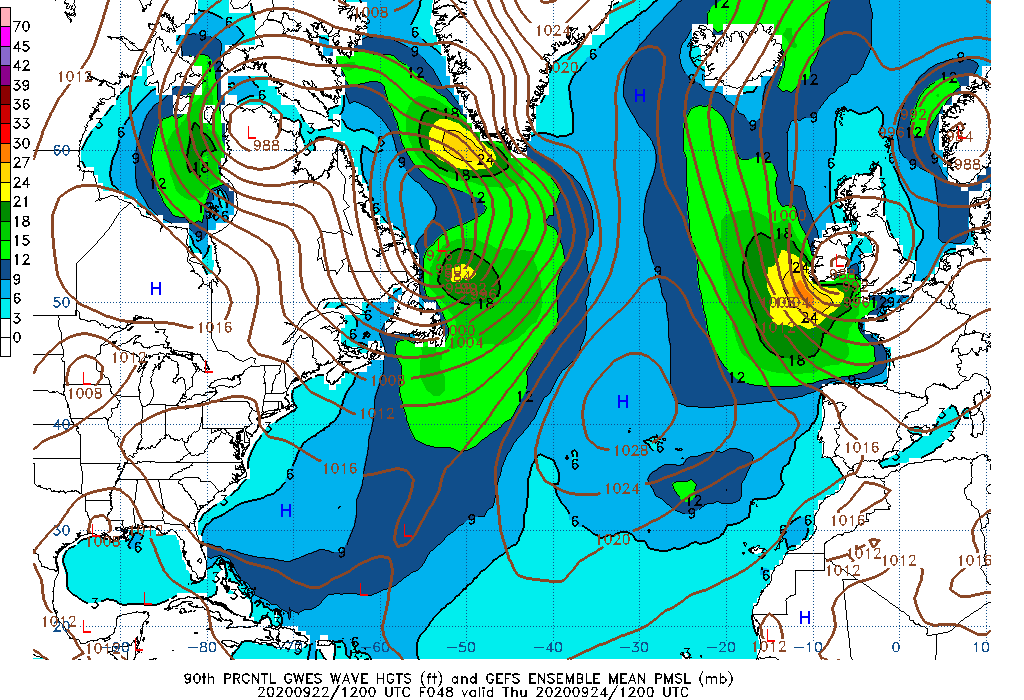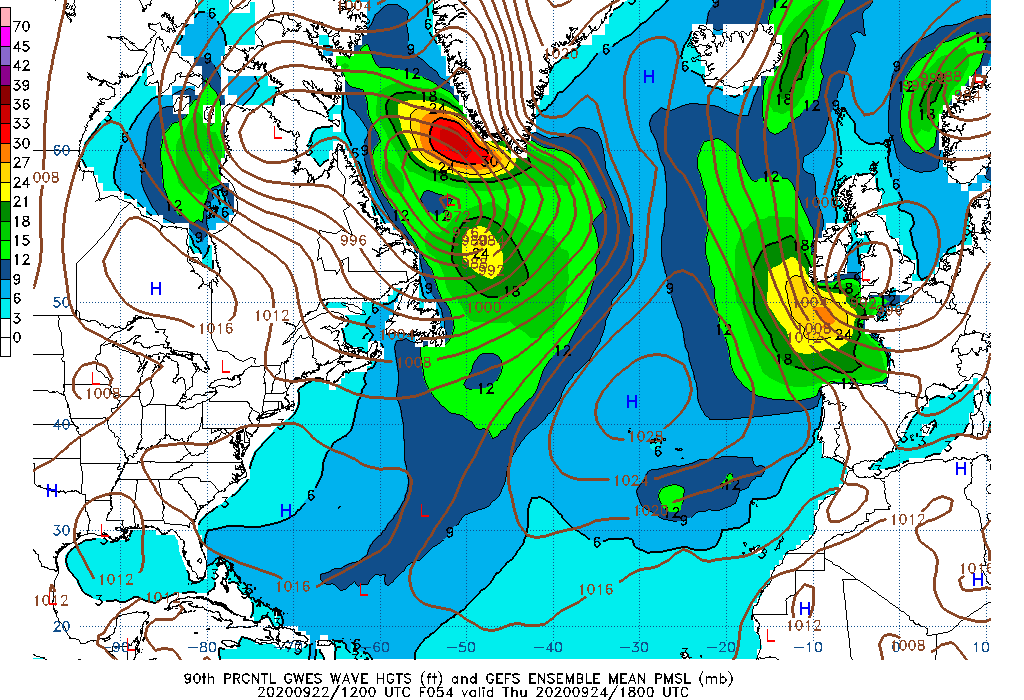048 Hour Wave Height 90th Percentile 054 Hour Wave Height 90th Percentile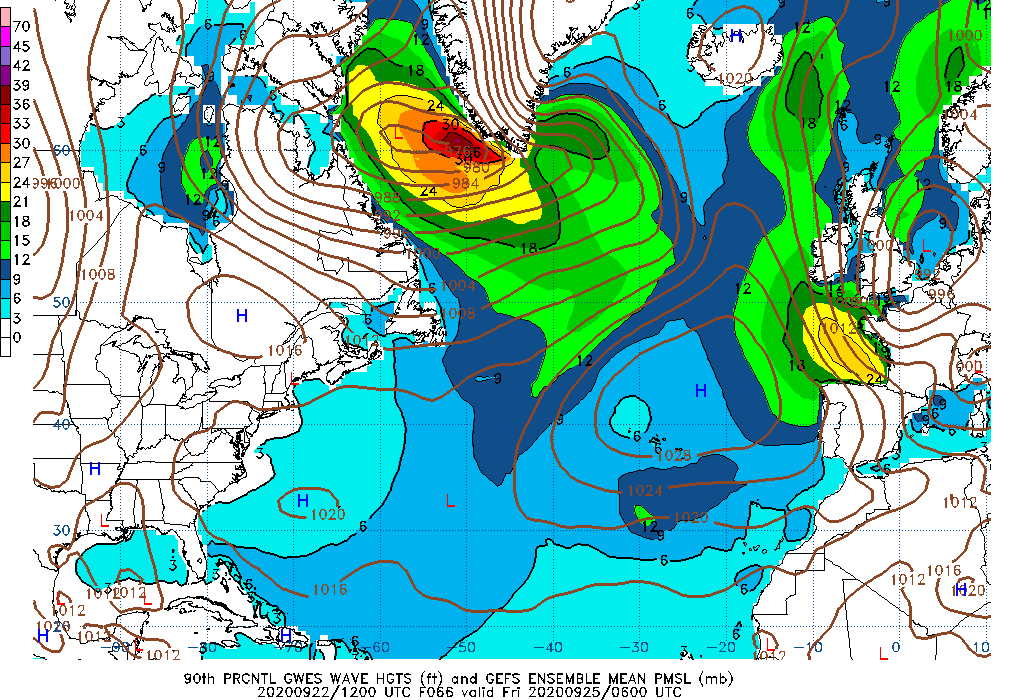060 Hour Wave Height 90th Percentile 066 Hour Wave Height 90th Percentile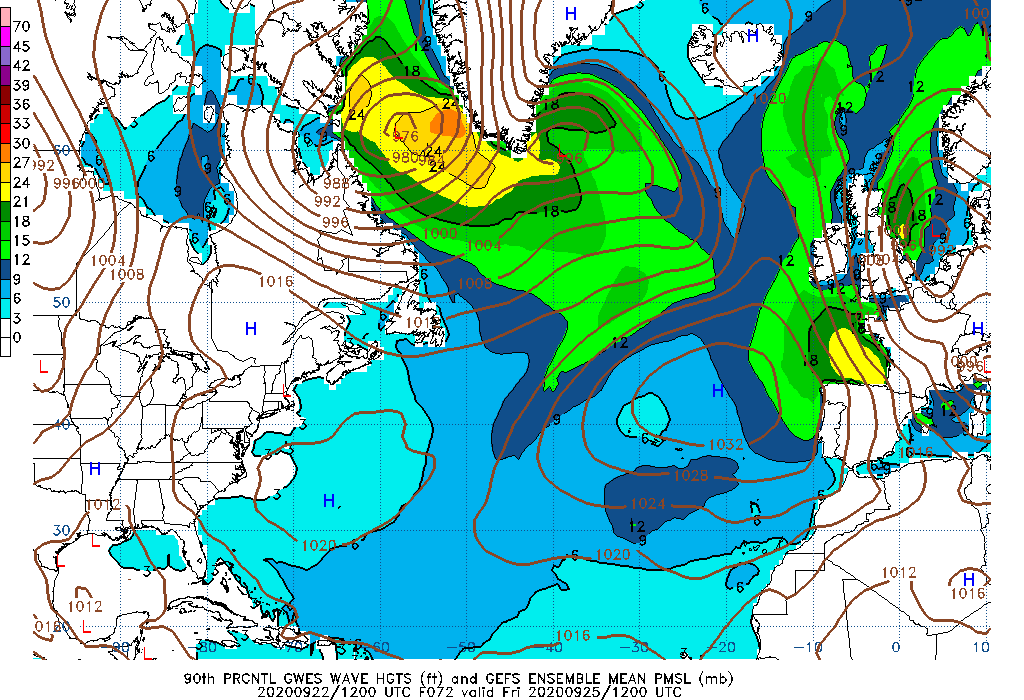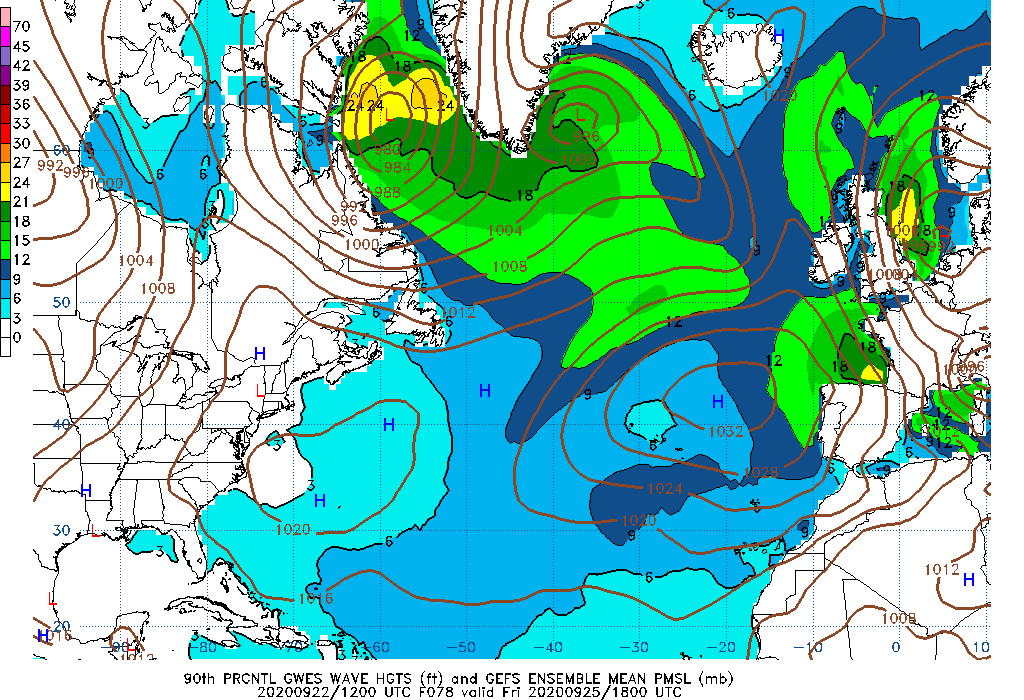072 Hour Wave Height 90th Percentile 078 Hour Wave Height 90th Percentile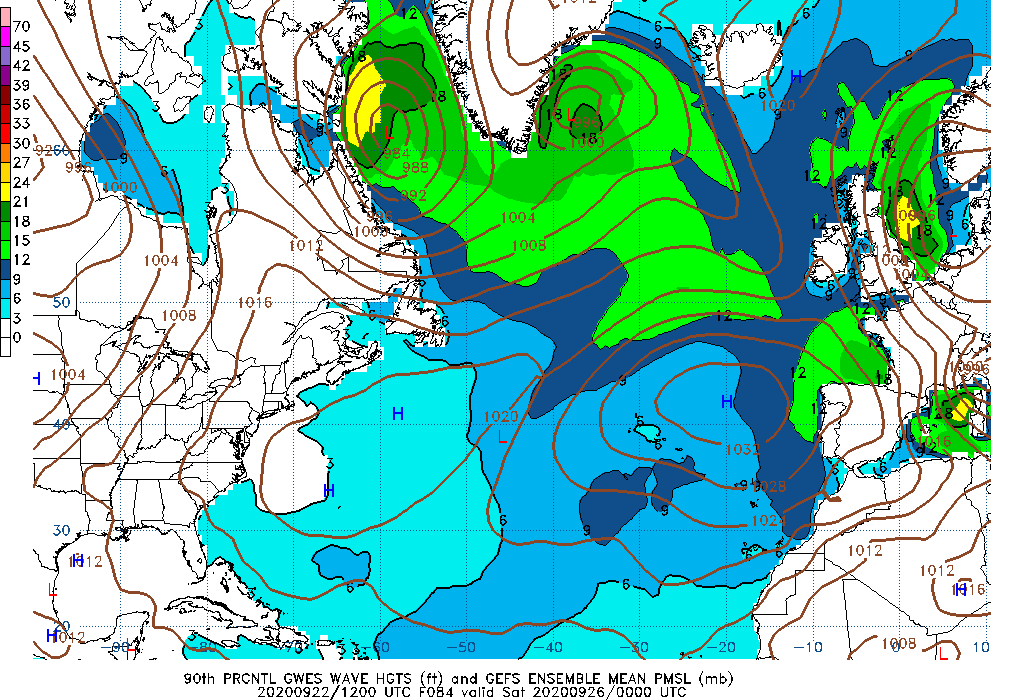084 Hour Wave Height 90th Percentile 090 Hour Wave Height 90th Percentile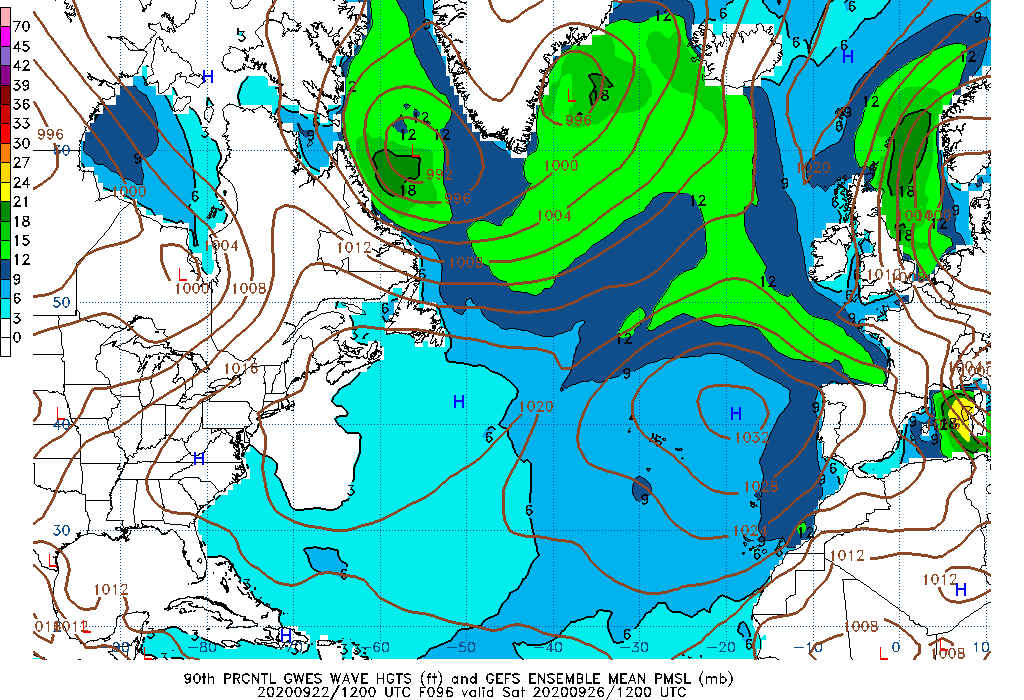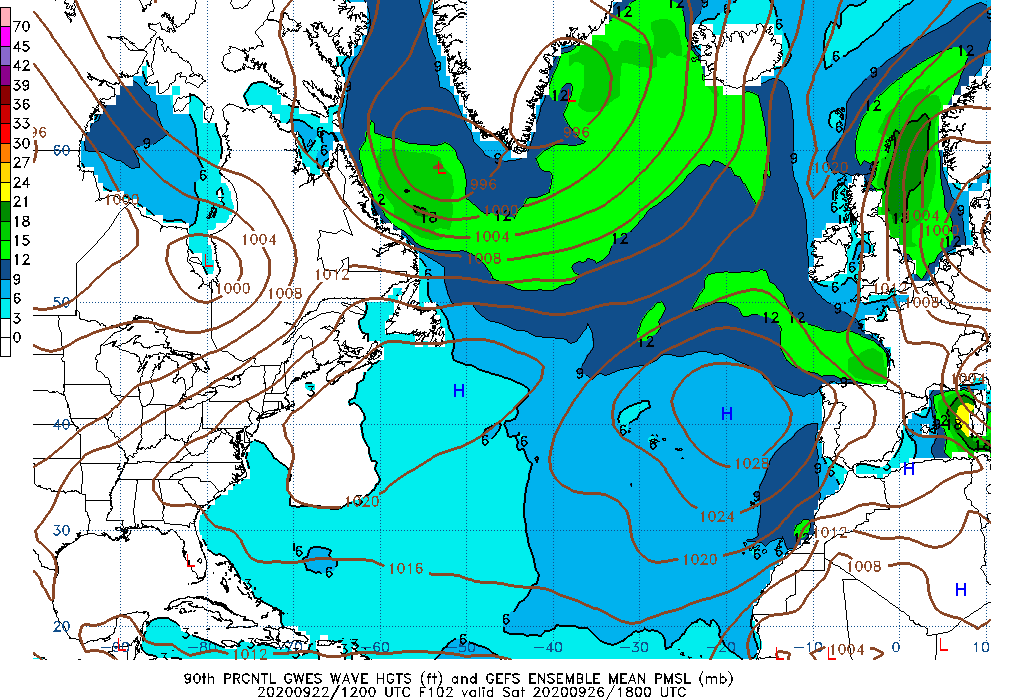096 Hour Wave Height 90th Percentile 102 Hour Wave Height 90th Percentile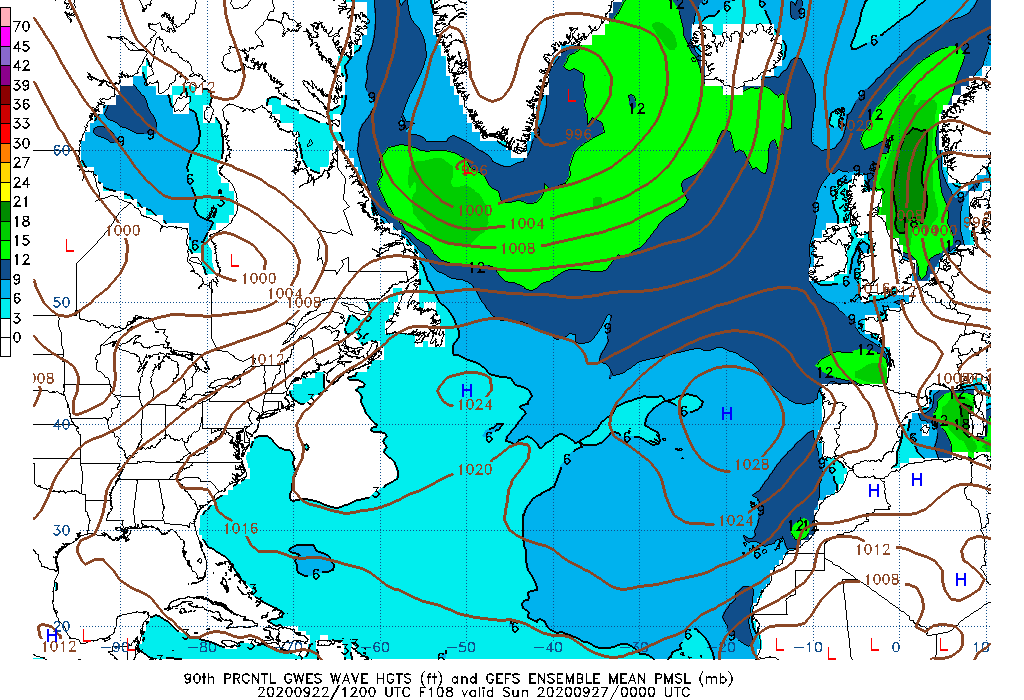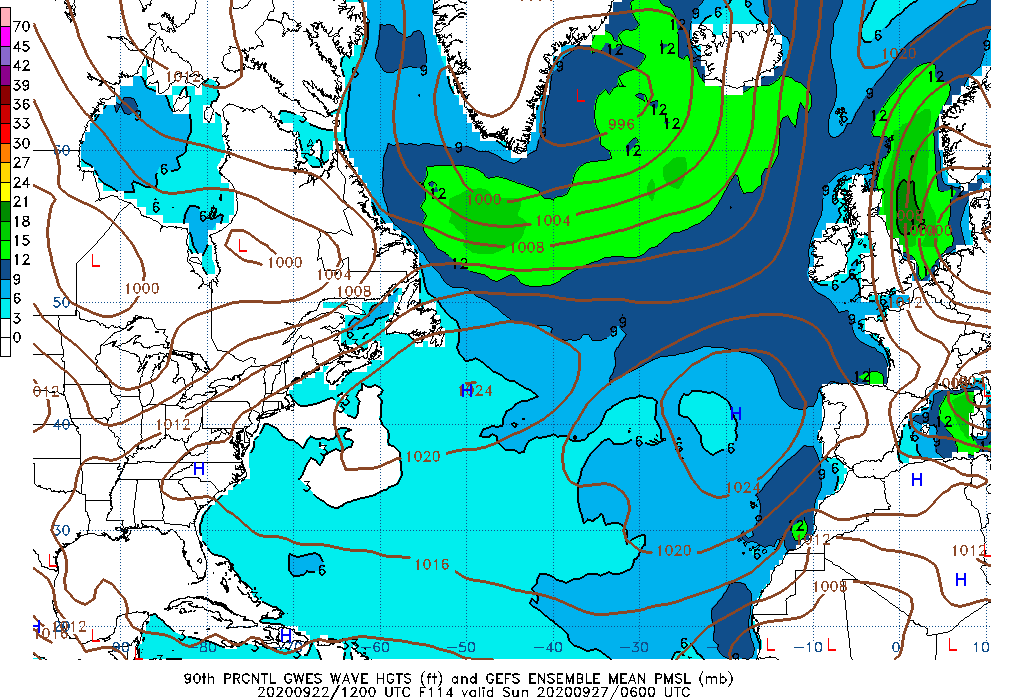108 Hour Wave Height 90th Percentile 114 Hour Wave Height 90th Percentile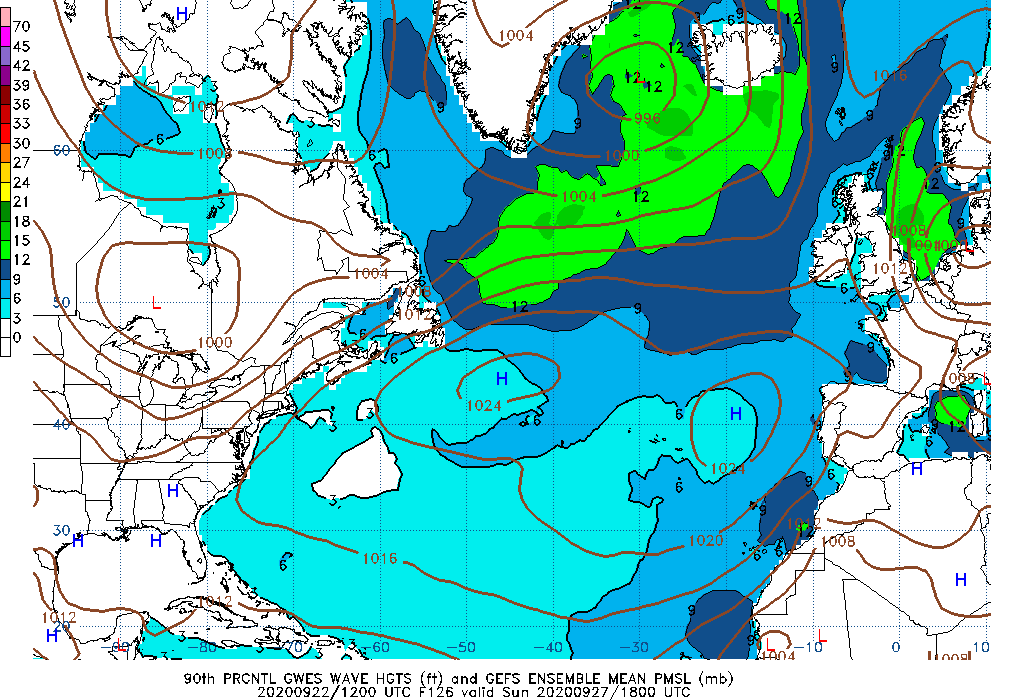120 Hour Wave Height 90th Percentile 126 Hour Wave Height 90th Percentile132 Hour Wave Height 90th Percentile 138 Hour Wave Height 90th Percentile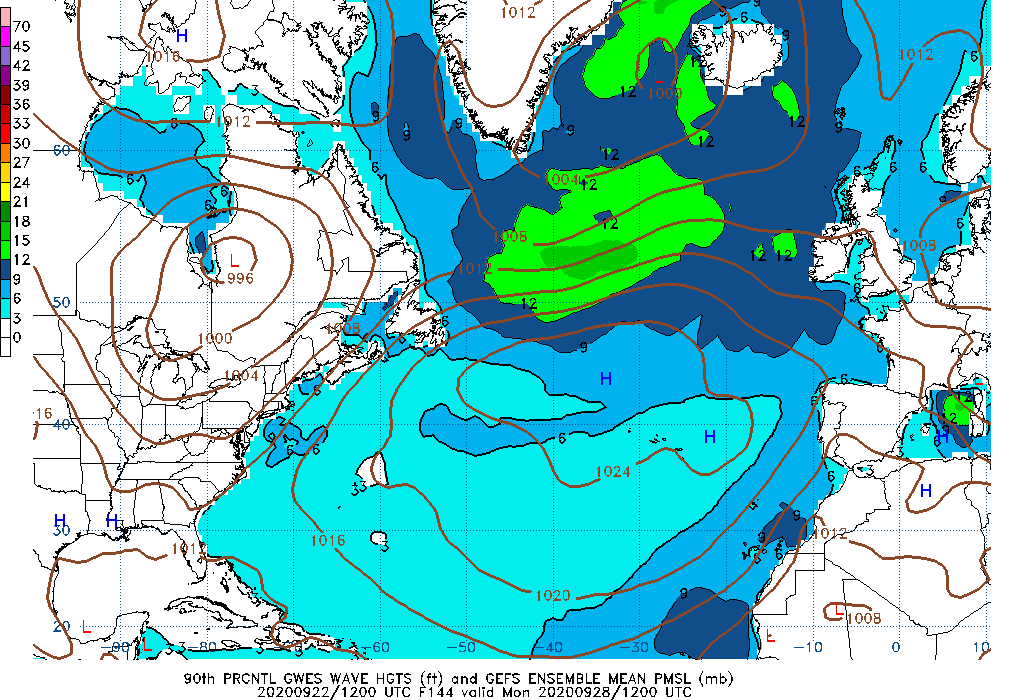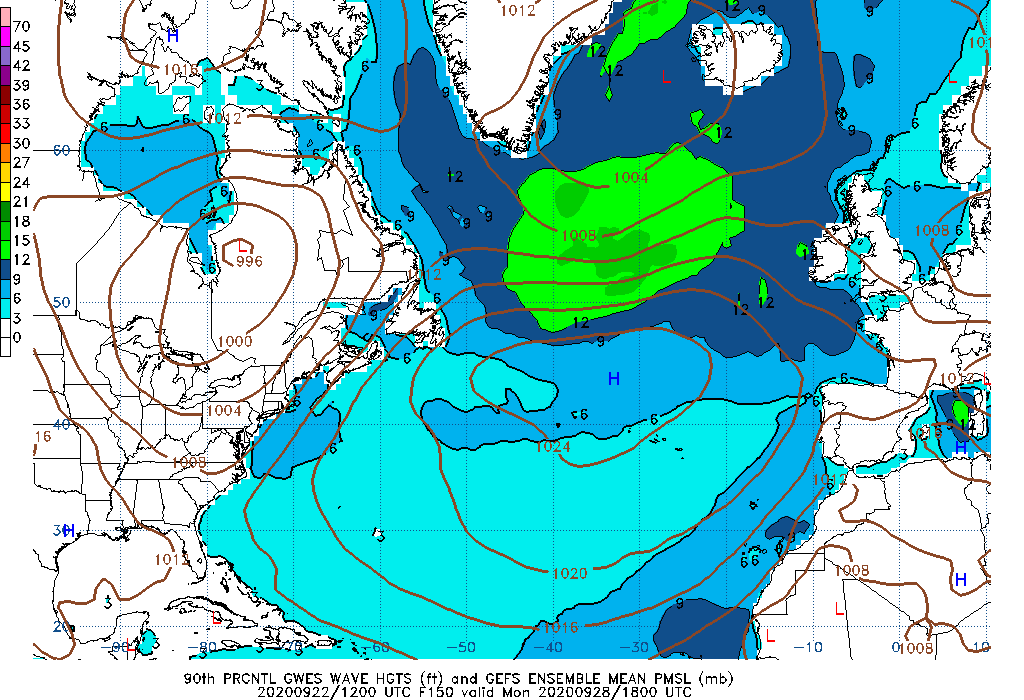144 Hour Wave Height 90th Percentile 150 Hour Wave Height 90th Percentile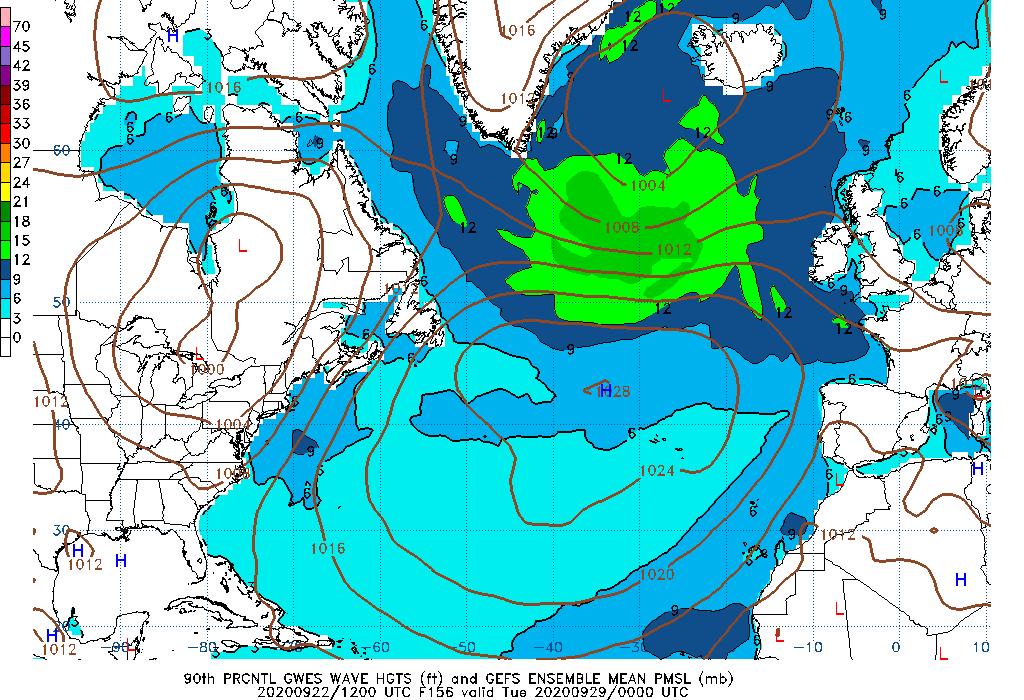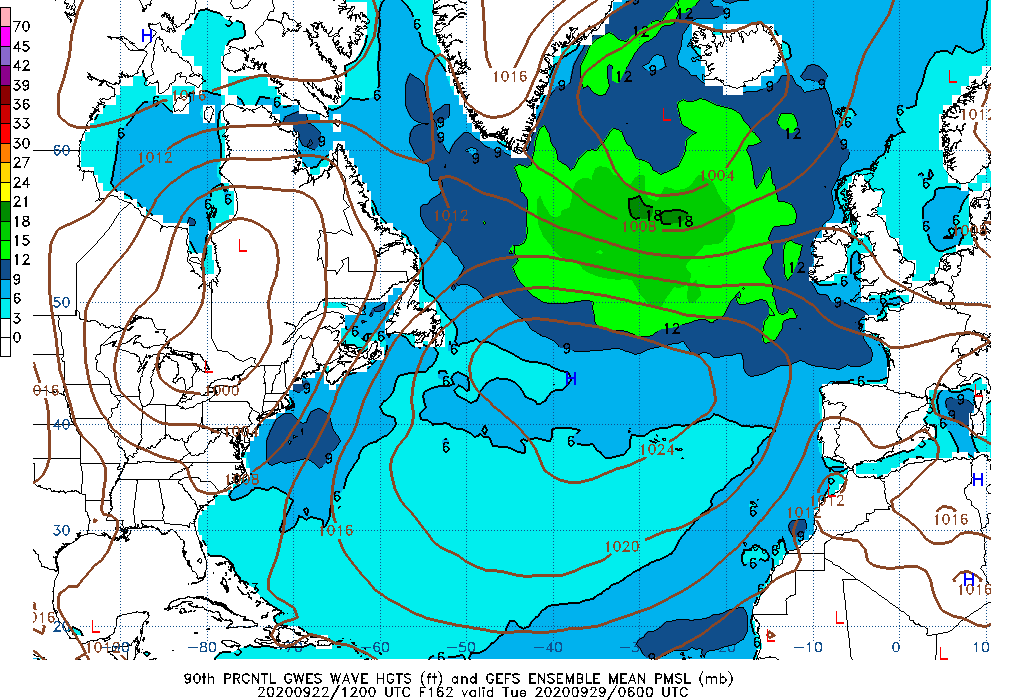156 Hour Wave Height 90th Percentile 162 Hour Wave Height 90th Percentile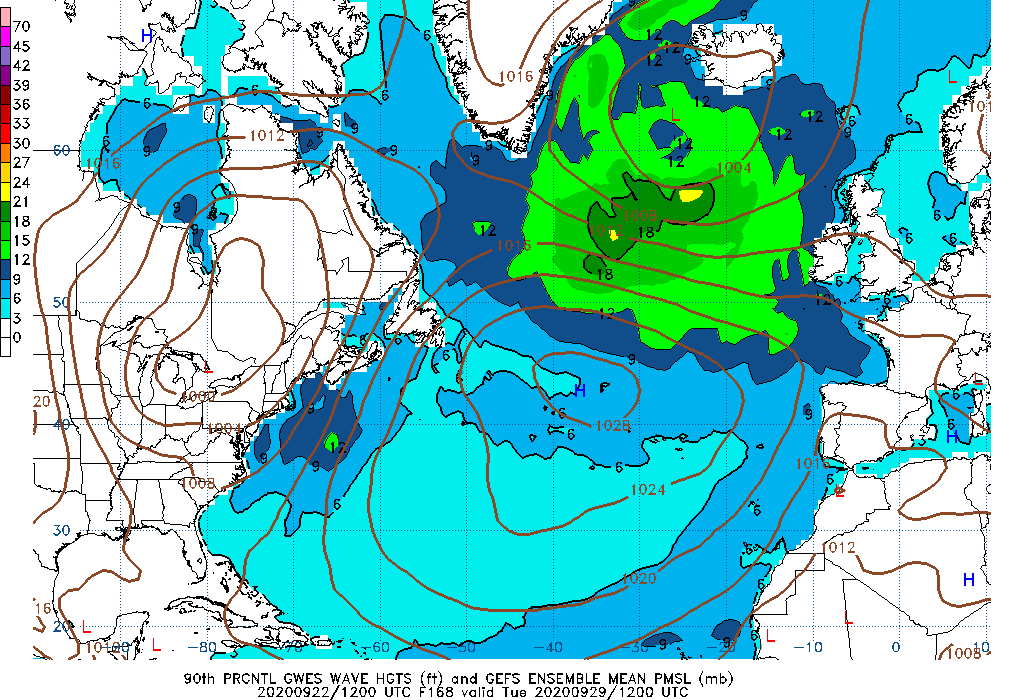168 Hour Wave Height 90th Percentile 174 Hour Wave Height 90th Percentile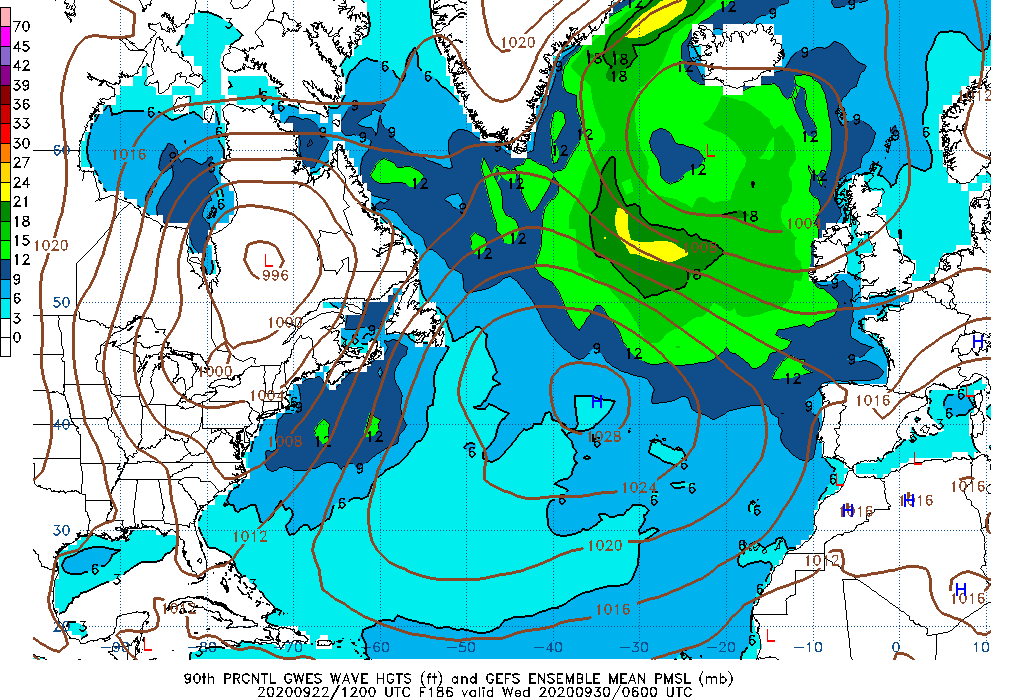180 Hour Wave Height 90th Percentile 186 Hour Wave Height 90th Percentile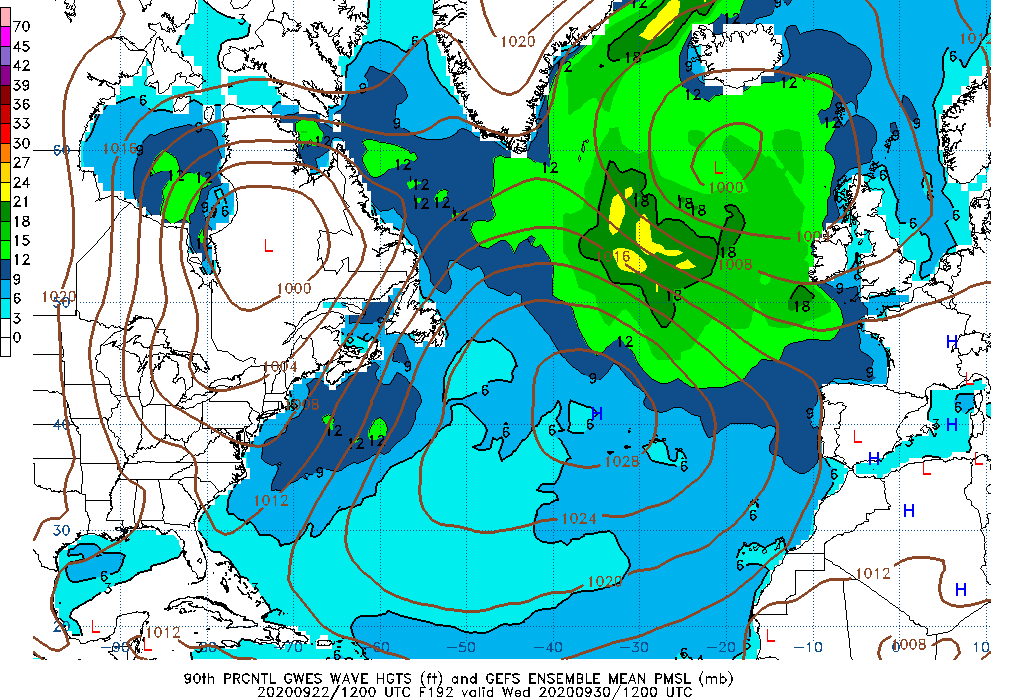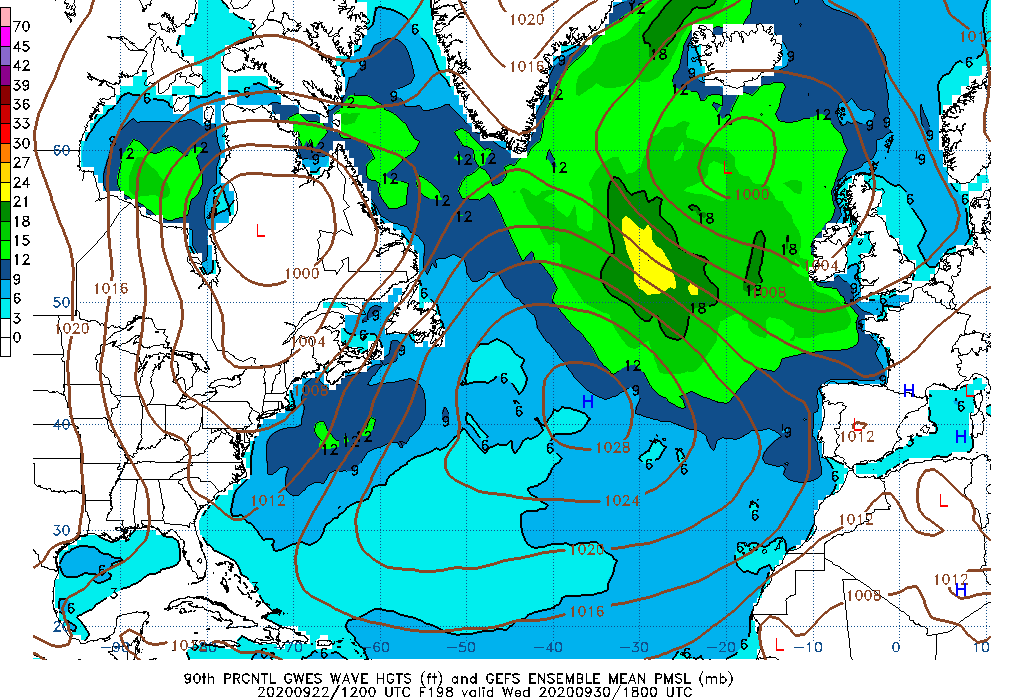192 Hour Wave Height 90th Percentile 198 Hour Wave Height 90th Percentile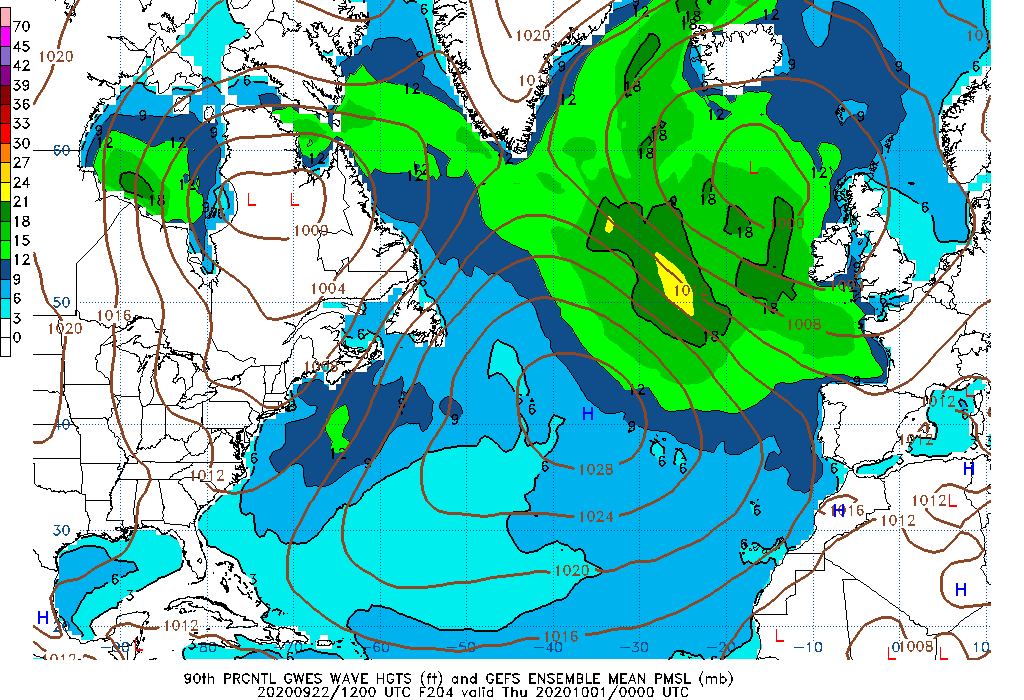204 Hour Wave Height 90th Percentile 210 Hour Wave Height 90th Percentile216 Hour Wave Height 90th Percentile 222 Hour Wave Height 90th Percentile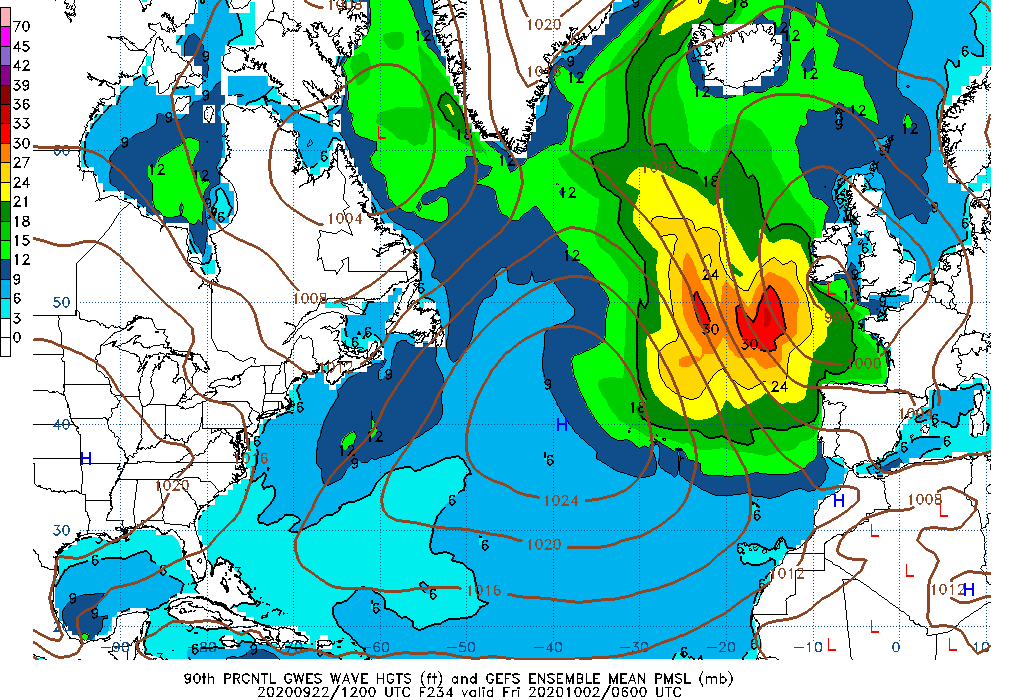228 Hour Wave Height 90th Percentile 234 Hour Wave Height 90th Percentile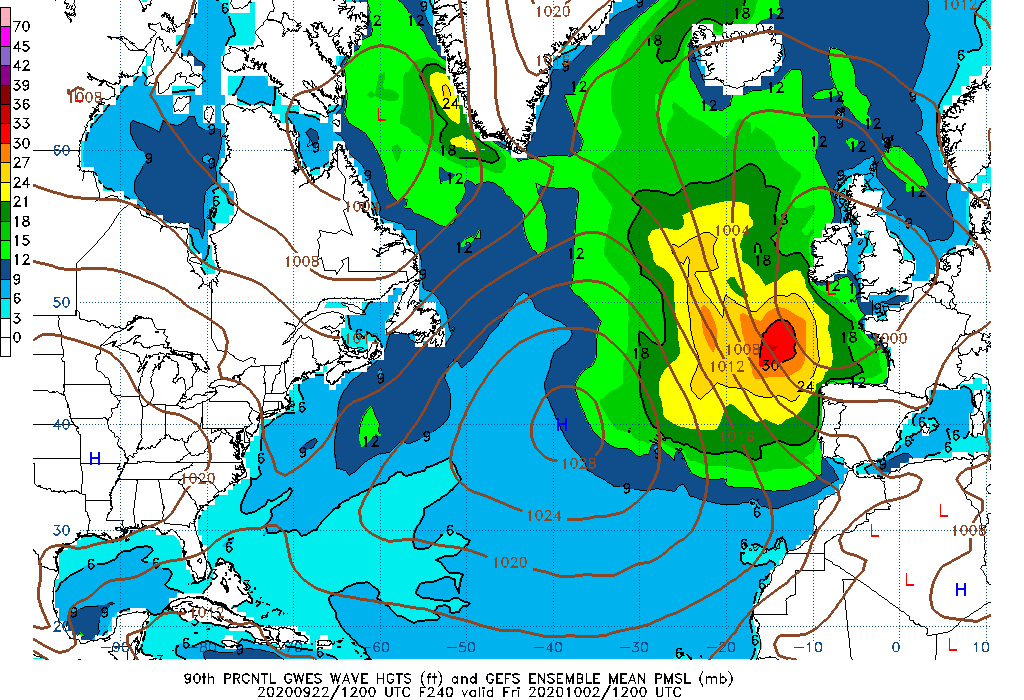240 Hour Wave Height 90th Percentile

Notes on images above:

• The likelihood of exceedance is shown as a probability of 0 to 100 of waves heights (significant wave height) reaching or being greater than the chosen value.

• The 90th percentile represents the wave height value that 90% of the ensemble members are either less than or equal to (i.e., 18 feet).

• The 10th percentile is the wave height value that is represented by 10% of the contributing ensemble members.

• The mean is defined as the 50th percentile, that is, the wave height value represented reflects half the distribution is above and half is below this value.

• The maximum value is the highest value produced by any of the 21 ensemble members. It reflects the most severe conditions predicted by the ensemble prediction system.

The data are produced from the Global Ensemble Forecast System (GEFS) which is run every 6 hours and are created with 20 ensemble members.

Additional information for interpreting this data